# Ito process

An Ito process is a type of stochastic process described by Japanese mathematician Kiyoshi Itô, which can be written as the sum of the integral of a process over time and of another process over a Brownian motion. Those processes are the base of Stochastic integration, and are therefore widely used in financial mathematics and stochastic calculus.

## Description of the Ito Processes

Let$(\Omega, \mathcal{F}, \mathbb{F}, \mathbb{P})$ be a probability space with a filtration$\mathbb{F}=(\mathcal{F}_t)_{t\geq 0}$ that we consider as complete (that is to say, all sets which measure is equal to zero are contained in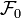$\mathcal{F}_0$). Let also be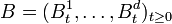$B=(B^1_t,\dots,B^d_t)_{t\geq 0}$ a d-dimensional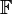$\mathbb{F}$-standard Brownian motion. Then we call "Ito process" every process$(X_t)_{t\geq 0}$ that can be written in the form$X_t = X_0 + \int_0^t K_s\mathrm{ds} + \sum_{j=1}^d\int_0^t H^j_s\mathrm{dB}_s^j,$

where

• X0 is$\mathcal{F}_0$ measurable,
•$(K_t)_{t\geq 0}$ is a progressively measurable process such that$\forall t\geq 0,\; \int_0^t|K_s|\textrm{ds}<+\infty$ almost surely.
•$(H^i_t)_{t\geq 0,\ i\in[1\dots d]}$ is progressively measurable and such as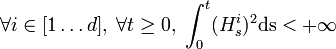$\forall i\in [1\dots d],\; \forall t\geq 0,\; \int_0^t(H_s^i)^2\mathrm{ds}<+\infty$ almost surely.

We denote by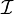$\mathcal{I}$ the set of all Ito processes. All Ito processes are continuous and adapted to the filtration$\mathbb{F}$. We can also write the Ito process in the 'differential form':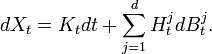$dX_t = K_tdt + \sum_{j=1}^dH_t^jdB_t^j.$

Using the fact that the Brownian part is a local martingal, and that all continuous local martingal with finite variations equal to zero in zero is indistinguishible from the null process, we can show that this decomposition is unique (up to indistinguishability) for each Ito process.

## Stochastic Integral with respect to an Ito process

Let X be an Ito process. We can define the set of processes$\mathcal{L}(X)$ that we can integrate with respect to X: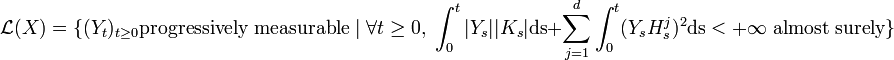$\mathcal{L}(X) = \{(Y_t)_{t\geq 0} \mathrm{progressively}\; \mathrm{measurable}\; |\; \forall t\geq 0,\; \int_0^t|Y_s||K_s|\textrm{ds} + \sum_{j=1}^d\int_0^t (Y_sH_s^j)^2\textrm{ds} < +\infty\; \textrm{almost}\; \mathrm{surely}\}$

We can then write: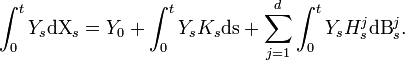$\int_0^tY_s\textrm{dX}_s = Y_0 + \int_0^t Y_sK_s\mathrm{ds} + \sum_{j=1}^d\int_0^t Y_sH^j_s\mathrm{dB}_s^j.$

### Stability of the set of Ito processes

The sum of two Ito processes is obviously another Ito process.
Stability over Integration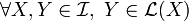$\forall X,Y\in \mathcal{I},\ Y\in \mathcal{L}(X)$. Which means that any Ito process can be integrated with respect to any other Ito process. Moreover, the stochastic integral with respect to an Ito process is still an Ito process.

This exceptional stability is one of the reasons of the wide use of Ito processes. The other reason is the Ito formula.

### Quadratic Variation of an Ito Process

Let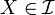$X\in\mathcal{I}$. The construction of the stochastic integral makes the usual formula for deterministic functions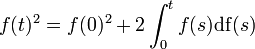$f(t)^2 = f(0)^2 + 2\int_0^tf(s)\mathrm{df}(s)$ wrong for the Ito processes. We then define the quadratic variation as the process < X,X > t:$<\!X,X\!>_t = X_t^2 - X_0^2 - 2\int_0^tX_s\mathrm{dX}_s$

This process is adapted, continuous, equal to zero in zero, and its trajectories are almost surely increasing.

We can define similarly the covariation of two Ito processes :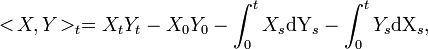$<\!X,Y\!>_t = X_tY_t - X_0Y_0 - \int_0^tX_s\mathrm{dY}_s - \int_0^tY_s\mathrm{dX}_s,$

which is also adapted, continuous, equal to zero in zero, and has finite variation.
We can then note the following properties of the quadratic variation:

1. If X or Y has finite variation then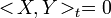$<\!X,Y\!>_t = 0$
2. If$M,N\in\mathcal{I}$ are local martingales then$<\!M,N\!>_t$ is the only adapted, continuous, vanishing at zero process of finite variation such that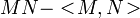$MN-<\!M,N\!>$ is a local martingale.
3. If we have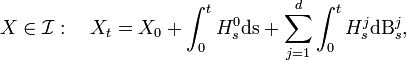$X\in\mathcal{I}:\quad X_t = X_0 + \int_0^t H^0_s\mathrm{ds} + \sum_{j=1}^d\int_0^t H^j_s\mathrm{dB}_s^j,$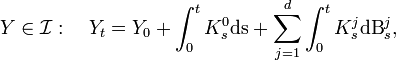$Y\in\mathcal{I}:\quad Y_t = Y_0 + \int_0^t K^0_s\mathrm{ds} + \sum_{j=1}^d\int_0^t K^j_s\mathrm{dB}_s^j,$

then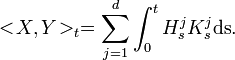$<\!X,Y\!>_t = \sum_{j=1}^d\int_0^t H^j_sK^j_s\mathrm{ds}.$Some content on this page may previously have appeared on Citizendium.